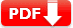# Exponents Practice Test Question Answers

Exponents are used to expressing large numbers in the shorter forms to make them easy to read, understand, compare, and operate upon.  a × a × a × a = a4 (read as ‘a’ raised to the exponent 4 or the fourth power of a), where ‘a’ is the base and 4 is the exponent and a4 is called the exponential form. a × a × a × a is called the expanded form.

 Test Name Exponents & Powers Test Prep Type of Question Multiple Choice Question Answers Subject Math Total Question 10 Test Type Sample / Mock Test Editable & Printable PDF / Doc YES (Download link is given below) Available of Answers YES Difficulty Level Pre-Algebra

### Exponents Practice Test Question Answers

SET: 1

 1.  Out of the following, the number which is not equal to (– 8/27) is 🔘 A. – (¾)3 🔘 B. – (½)3 🔘 C. – (2/3)3 🔘 D. (2/3)3 🔘 E. (-2/3)3
 2. (-7)5 × (-7)3 is equal to 🔘 A. (-7)5 🔘 B. (7)2 🔘 C. (-7)-5 🔘 D. 7 🔘 E. (-7)8
 3. Which one of the following exponential notation of 648? 🔘 A. 32 × 43 🔘 B. 23 × 34 🔘 C. 33 × 44 🔘 D. 23 × 43 🔘 E. 33 × 33
Answer: B 23 × 3 (648 = 2 × 2 × 2 × 3 × 3 × 3 × 3)
 4. What is the value of x ? So that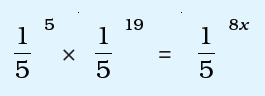🔘 A. 1 🔘 B. 2 🔘 C. 3 🔘 D. 4 🔘 E. 5
Answer: C 3 (formula am× an = am+n
 5. If 21998 – 21997 – 21996 + 21995 = E21995 then the value of E is 🔘 A. 1 🔘 B. 2 🔘 C. 3 🔘 D. 4 🔘 E. 11
Answer: C E=3 (hints: cross multiplication)
 6. Which of the following is not equal to 1 ? 🔘 A.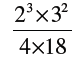🔘 B.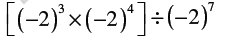🔘 C.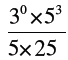🔘 D.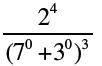🔘 E. NOTA
 7. Choose one of the following answer to make the statements true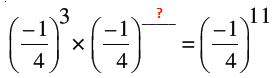🔘 A. (-1/4)2 🔘 B. (-1/4)3 🔘 C. (-1/4)4 🔘 D. (-1/4)8 🔘 E. (-1/4)9
 8. Choose one of the following answer to make the statements true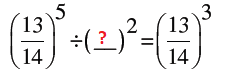🔘 A. (14/15) 🔘 B. (13/14) 🔘 C. (13/15) 🔘 D. (12/13) 🔘 E. (14/16)
 Document Type Download Link Free Editable Doc File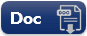Free Printable PDF File